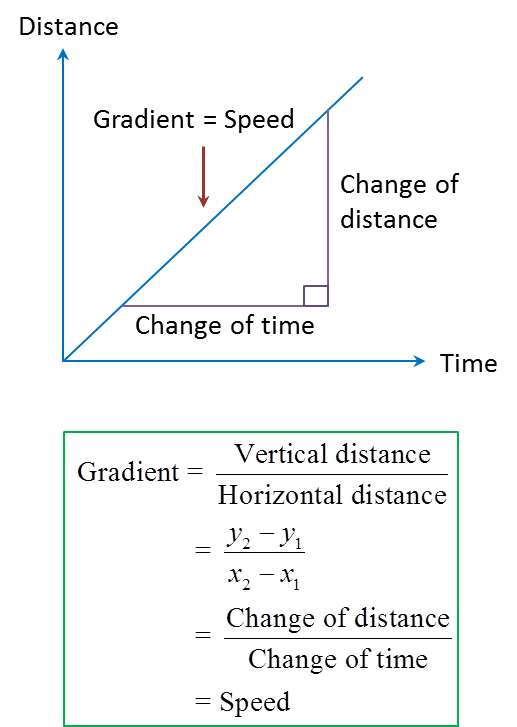# 6.1.1 Quantity Represented by the Gradient of a Graph (Part 1)

6.1 Quantity Represented by the Gradient of a Graph
The gradient of graph is the rate of change of a quantity on the vertical axis with respect to the change of another quantity on the horizontal axis.

(A) Distance – Time Graph
1. The gradient of a distance-time graph is speed.2.(a) From O to P: Gradient = positive
(b) From P to Q: Gradient = 0 → (The object stopped moving)
(c) From Q to R: Gradient = negative → (The object is travelling in an opposite direction from the original direction).

3.Example 1:

The diagram above shows a graph of distance-time for the motion of a car. Find the speed of the car for the first 6 second.

Solution:
The speed of the car for the first 6 seconds
$\begin{array}{l}=\frac{12-0}{6-0}\\ =2.0{\text{ms}}^{-1}\end{array}$

### 3 thoughts on “6.1.1 Quantity Represented by the Gradient of a Graph (Part 1)”

1.I hate maths 😊

2.Sorry typo i love maths

•🙂